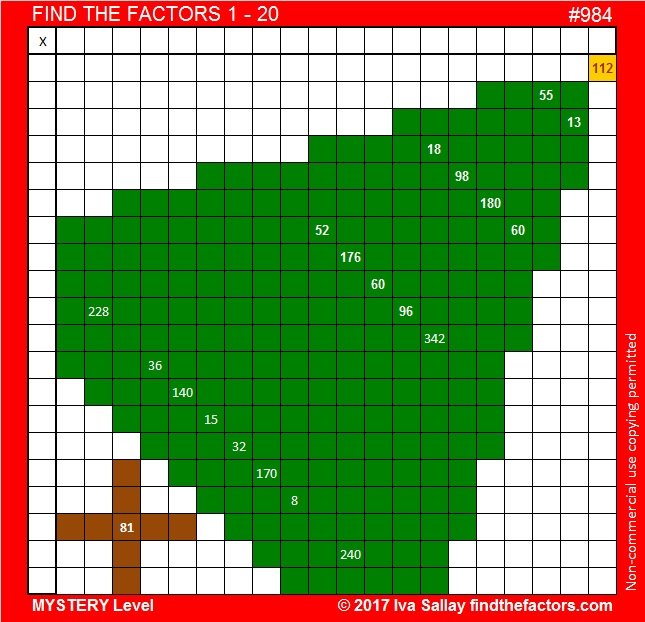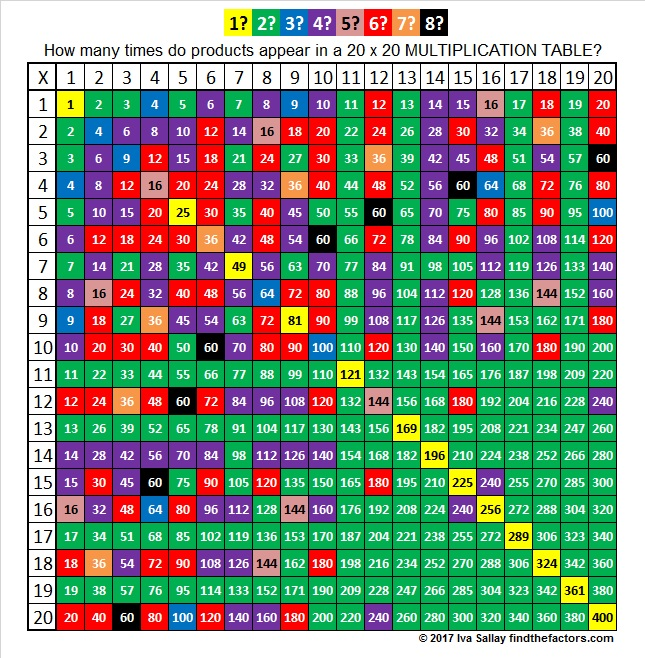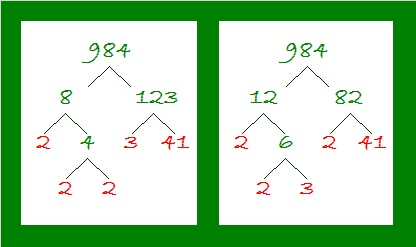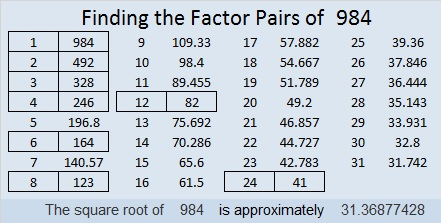# 984 Way Too Big Christmas Factor Tree

Some Christmas trees are so big they are difficult to take home in the car. They might even be too big to set up in the house. This puzzle is the biggest one I have ever made. It looks like a very big Christmas tree waiting to be set up. Is it too big to bring fun this Christmastime?The table below may be helpful in solving the puzzle. There are 400 places to write products in a 20 × 20 multiplication table, but not all the numbers from 1 to 400 appear in such a table. Some numbers don’t appear at all while other numbers appear more than one times. The chart below is color-coded to show how many times a product appears in the 20 × 20 multiplication table. Clues in the puzzle that appear only once (yellow) or twice (green) in the multiplication table won’t cause much trouble when solving the puzzle. Any other clues might stump you. Notice that the number 60 appears twice in the puzzle but eight times (black) in the 20 × 20 multiplication table!I’d like to share some information about the number 984.

It is the hypotenuse of a Pythagorean triple:
216-960-984 which is 24 times (9-40-41)

OEIS.org informs us that 8 + 88 + 888 = 984.

984 is 1313 in BASE 9 because 1(9³) + 3(9²) + 1(9¹) + 3(9⁰) = 984.

Here are a couple of the many possible factor trees for 984:• 984 is a composite number.
• Prime factorization: 984 = 2 × 2 × 2 × 3 × 41, which can be written 984 = 2³ × 3 × 41
• The exponents in the prime factorization are 3, 1, and 1. Adding one to each and multiplying we get (3 + 1)(1 + 1)(1 + 1) = 4 × 2 × 2 = 16. Therefore 984 has exactly 16 factors.
• Factors of 984: 1, 2, 3, 4, 6, 8, 12, 24, 41, 82, 123, 164, 246, 328, 492, 984
• Factor pairs: 984 = 1 × 984, 2 × 492, 3 × 328, 4 × 246, 6 × 164, 8 × 123, 12 × 82, or 24 × 41
• Taking the factor pair with the largest square number factor, we get √984 = (√4)(√246) = 2√246 ≈ 31.36877This site uses Akismet to reduce spam. Learn how your comment data is processed.